# 神经网络实现手写数字识别（MNIST）

## 二、人工神经网络简介

### 2.1 生物学神经网络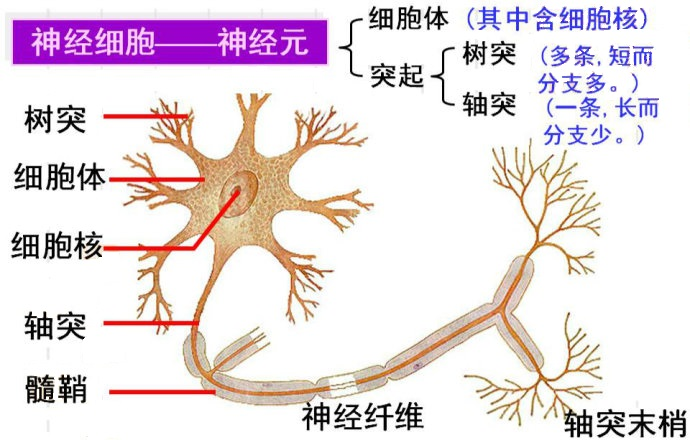• 轴突——树突——细胞体
• 轴突——细胞体——树突
• 树突——细胞体——轴突

### 2.2 人工神经网络——神经细胞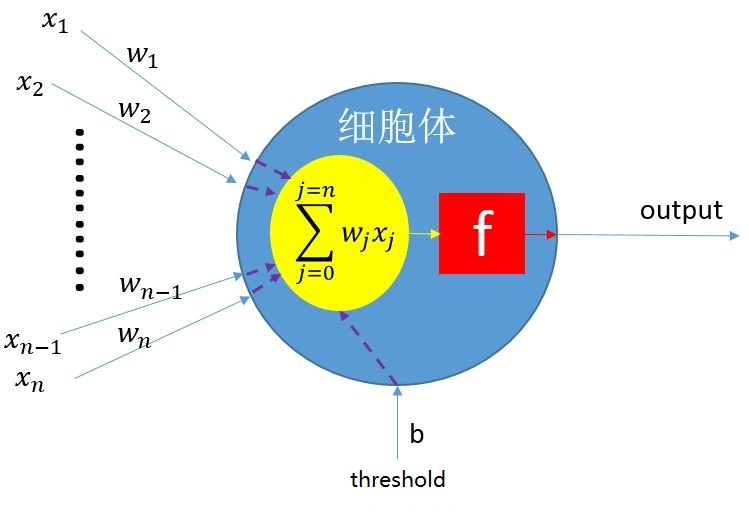• x1 … xn：表示神经细胞的输入，也就是输入神经细胞的信号。
• w1 … wn：表示每个输入的权重，就好比生物神经网络中每个轴突和树突的连接的粗细，强弱的差异。
• b：偏置权重
• threshold: 偏置（可以将 threshold * b 看作是前面提到的生物神经细胞的阈值）
• 蓝色部分：细胞体。
• 黄色球形是所有输入信号以的求和。
• 红色部分是表示求和之后的信号的激励函数（即达到阈值就处于兴奋状态，反之抑制，当然作为人工神经细胞，其激励函数很多，阶跃（型）激励函数，sigmoid（s型）激励函数，双曲正切（tanh）激励函数，ReLu（Rectified Linear Units）激励函数等等）。

o u t p u t = { 0 i f ∑ j w j x j &lt; t h r e s h o l d 1 i f ∑ j w j x j ≥ t h r e s h o l d output=\begin{cases} 0 &amp;if \sum_{j} w_jx_j &lt; threshold \\ 1 &amp;if \sum_{j} w_jx_j \geq threshold \end{cases}

w 1 x 1 + w 2 x 2 + w 3 x 3 + ⋯ + w n x n ≥ t w_1x_1+w_2x_2+w_3x_3+\cdots+w_nx_n \geq t

w 1 x 1 + w 2 x 2 + w 3 x 3 + ⋯ + w n x n − t ≥ 0 w_1x_1+w_2x_2+w_3x_3+\cdots+w_nx_n - t\geq 0

w 1 x 1 + w 2 x 2 + w 3 x 3 + ⋯ + w n x n + t b ≥ 0 w_1x_1+w_2x_2+w_3x_3+\cdots+w_nx_n + tb\geq 0

w 1 x 1 + w 2 x 2 + w 3 x 3 + ⋯ + w n x n + w t x b ≥ 0 w_1x_1+w_2x_2+w_3x_3+\cdots+w_nx_n + w_tx_b\geq 0

### 2.3 人工神经网络——神经细胞层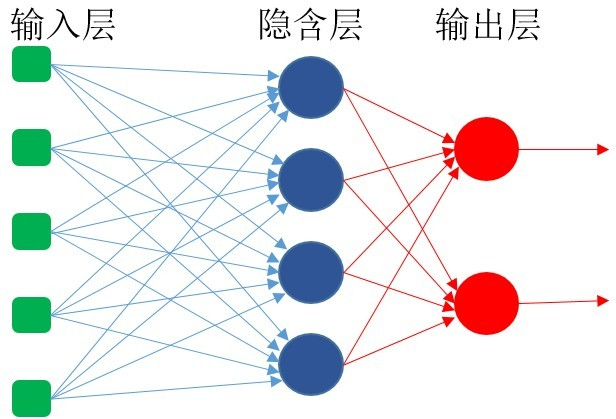### 2.4 人工神经网络——演化

f ( x ) = 1 1 + e ( − x ) f(x)=\frac{1}{1+e^{(-x)}}

f ( x ) ′ = f ( x ) ( 1 − f ( x ) ) f(x)&#x27;=f(x)(1-f(x))

f ( x ) = x ( 1 − x ) f(x)=x(1-x)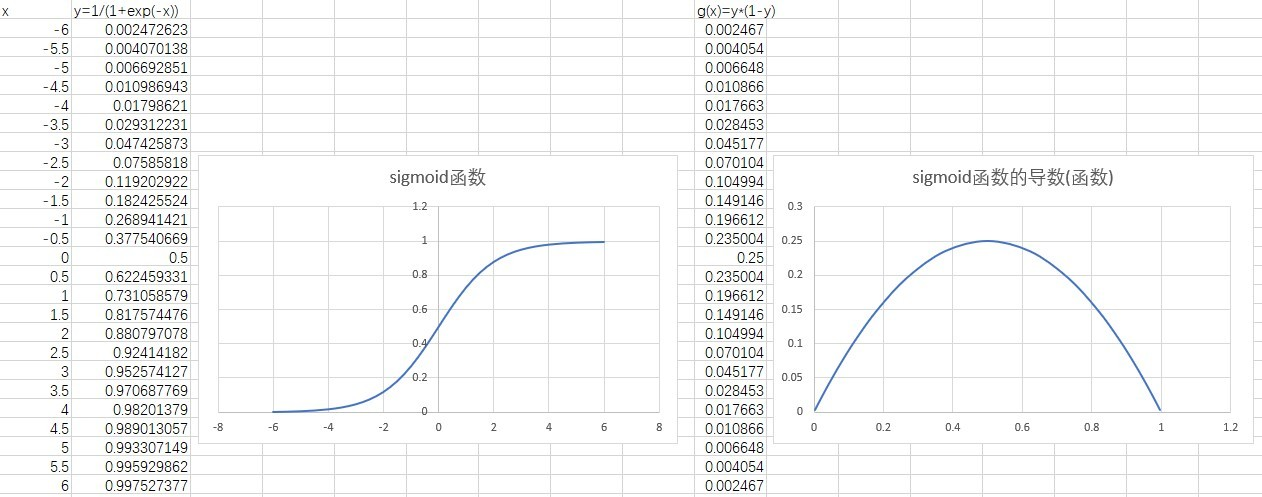Δ W n j = E n L O ( n − 1 ) j \Delta W_{nj}=E_nLO_{(n-1)j}

E = e   o ( 1 − o ) E=e \ o(1-o)

• 对于输出层：e = 当前神经细胞的输出 - 预期输出
• 对于隐含层：反向传播方向的上一层的所有神经细胞乘以与当前神经细胞的连接权重的累加和，其计算公式如下：

e = ∑ j = 0 j = k e ( n + 1 ) j w ( n + 1 ) j e=\sum_{j=0}^{j=k}e_{(n+1)j}w_{(n+1)j}

• 反向传播误差：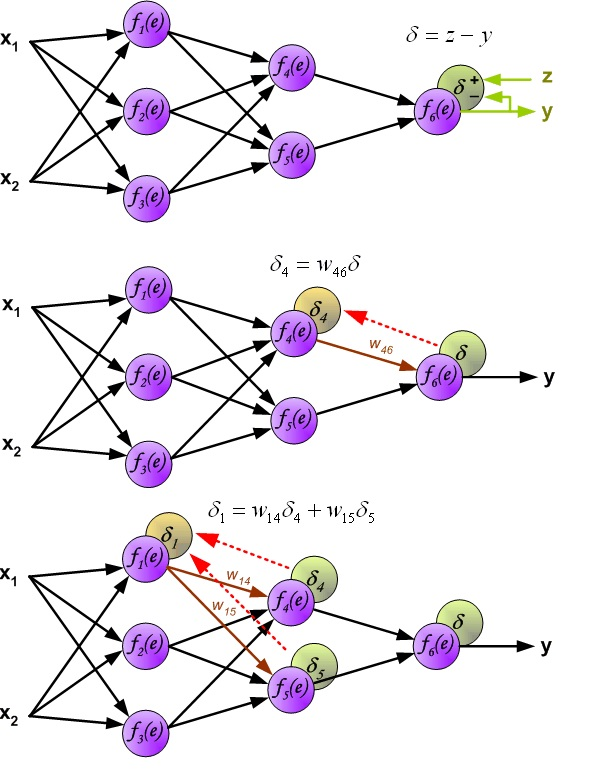• 更新权重（正向传播）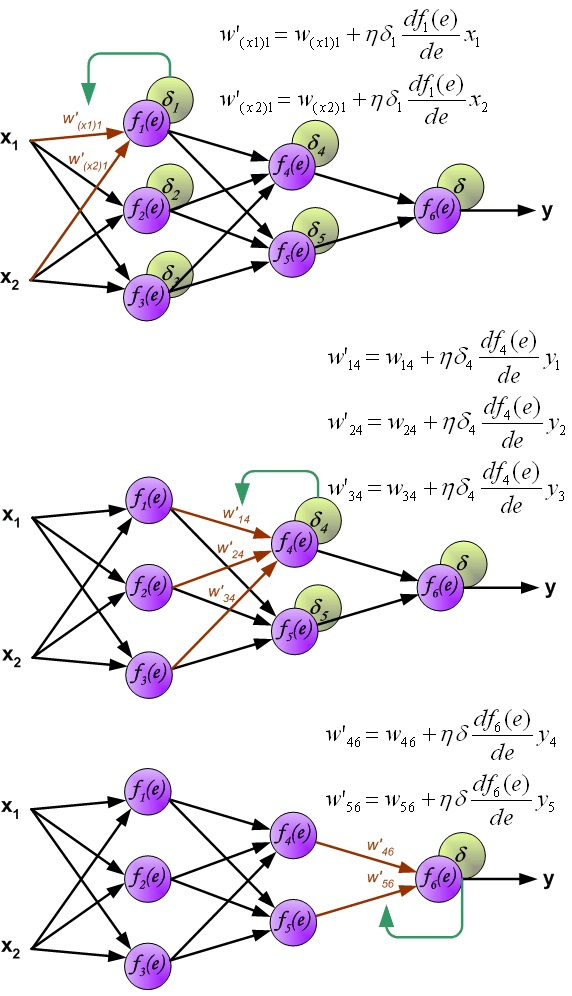## 三、手写数字识别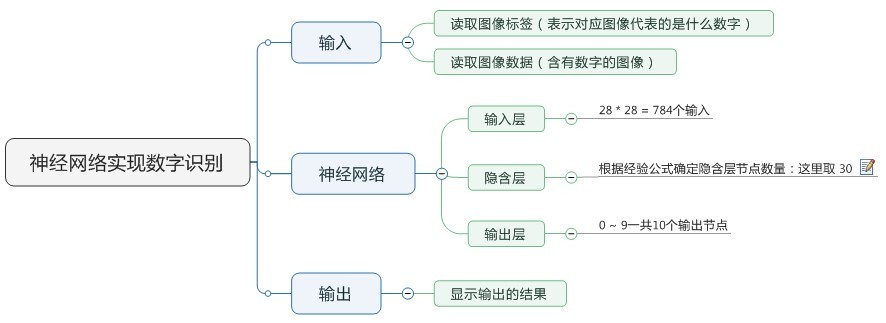m = n + l + a m=\sqrt{n+l}+a
m = log ⁡ 2 n m=\log_2n
m = n l m=\sqrt{nl}

• m: 隐含层节点数
• n: 输入层节点数
• l：输出层节点数
• a：1-10之间的常数

• 输入层节点n：784
• 输出层节点：10 （表示数字 0 ~ 9）

### 3.1 神经细胞，神经细胞层

• 输入权重数组
• 输入数目
• 输出值
• 误差值

struct neuron
{
//int mInputCount;     /** 当前神经细胞的输入数目 */
double mOutActivation; /** 当前神经细胞的输出 */
double mOutError;      /** 当前神经细胞的误差 */
vector<double> mWeights; /** 当前神经细胞的输入权重数组 */
};


struct neuronLayer
{
int mNumInputsPerNeuron; /** 当前层的每个神经细胞的输入数目 */
int mNumNeurons; /** 当前层的神经细胞数目 */
vector<neuron> mNeurons; /** 当前层的神经细胞数组 */
};


/** the type of neuron layer */

struct neuronLayer
{
public:
neuronLayer(int numNeurons, int numInputsPerNeuron); /** 神经细胞层的构造函数*/

neuronLayer(neuronLayer& nl); /** 神经细胞层的拷贝构造函数 */
~neuronLayer(); /** 神经细胞层的析构函数 */

void reset(void); /** 神经细胞层的重置函数（将权重等参数都重置为随机值）*/
public:
int mNumInputsPerNeuron; /** 当前层的每个神经细胞的输入数目 */
int mNumNeurons; /** 当前层的神经细胞数目 */
double** mWeights; /** 2维数组, 行: 代表神经细胞（每一行就是一个神经细胞的所有权重）, 列: 代表神经细胞的输入权重 */
double* mOutActivations; /** 当前层每个神经细胞的输出值 */
double* mOutErrors; /** 当前层每个神经细胞的误差值 */

};


### 3.2 神经网络

• 简单的全连接神经网络；
• 层数可自定义；
• 每一个隐含层的神经细胞数目可自定义；
• 使用反向传播以及梯度下降法进行网络演化；
• 激励函数使用：sigmoid（S型）函数；
• 使用c++语言实现。

class bpNeuronNet
{
public:
bpNeuronNet(int numInputs, double learningRate); /** 构造函数 */
~bpNeuronNet(); /** 析构函数 */
public:
/** 或者网络的总误差（输出层每个神经细胞输出误差的方差总和） */
inline double getError(void) { return mErrorSum; }

bool training(const double inputs[], const double targets[]); /** 训练网络 */
void process(const double inputs[], double* outputs[]); /** 处理数据（这里是数字识别） */

void reset(void); /** 重置网络 */
void addNeuronLayer(int numNeurons); /** 添加一个神经网络层 */

private:

/** 前向传播，计算网络的输出 */

/** sigmoid(S型)激活函数 */
inline double sigmoidActive(double activation, double response);

/** 更新一个神经网络层，计算其输出 */
void updateNeuronLayer(neuronLayer& nl, const double inputs[]);

/** 反向传播，训练网络 */

/** 反向传播的激活函数，sigmoid函数的导数 */
inline double backActive(double x);

/** 以训练模式更新网络，与 process 函数的区别是，这个函数会更新输出层的每个神经细胞的输出误差 */
void trainUpdate(const double inputs[], const double targets[]);

/** 训练一层神经细胞层 */
void trainNeuronLayer(neuronLayer& nl,  const double prevOutActivations[],
double prevOutErrors[]);

private:
int mNumInputs; /** 神经网络的输入数目 */
int mNumOutputs; /** 神经网络的输出数目 */
/** 隐含层数目，总的神经网络层的数目= mNumHiddenLayers + 1
* （不包含输入层，因为它是直接映射到第一个隐含层，没有神经细胞 ） */
int mNumHiddenLayers;
/** 神经网络的学习率（表示学习速度的），需要慎重选择，太大会出现错误收敛或者无法收敛，
* 太小也可能导致错误收敛，且学习速度变慢 */
double mLearningRate;
double mErrorSum; /** 忘了总误差，参看：getError函数 */
vector<neuronLayer*> mNeuronLayers; /** 神经网络包含的神经细胞层数组 */
};


• 比较简单的 sigmoid（S型）函数，公式前面已经说过了，代码实现如下：
double bpNeuronNet::sigmoidActive(double activation, double response)
{/** reponse 是用于缩放 activation的，取值范围： 0 < response <= 1.0 */
/** sigmoid function: f(x) = 1 /(1 + exp(-x)) */
return (1.0 / (1.0 + exp(-activation * response)));
}


• updateNeuronLayer函数，利用传入的inputs数据更新一个神经细胞层的输出数据：
void bpNeuronNet::updateNeuronLayer(neuronLayer& nl, const double inputs[])
{
int numNeurons = nl.mNumNeurons; /** 当前层有多少个神经细胞 */
int numInputsPerNeuron = nl.mNumInputsPerNeuron; /** 当前层的每一个神经细胞有多少输入 */
double* curOutActivations = nl.mOutActivations; /** 当前层所有神经细胞的输出值数组 */

/** 遍历每一个神经细胞 */
for (int n = 0; n < numNeurons; ++n)
{
double* curWeights = nl.mWeights[n]; /** 获取第n个神经细胞的输入权重数组 */

double netinput = 0;
int k;
/** 遍历每一个输入权重 */
for (k = 0; k < numInputsPerNeuron; ++k)
{
/*** 累加 weights 和 inputs的乘积 */
netinput += curWeights[k] * inputs[k];
}

/** 添加偏置项的值 */
netinput += curWeights[k] * BIAS;

/** 将累加后的值通过激活函数，得到当前神经细胞的最终输出 */
curOutActivations[n] = sigmoidActive(netinput, ACTIVATION_RESPONSE);
}
}



• process，数据处理函数，处理输入数据（本例是识别手写数字），得到相应输出
void bpNeuronNet::process(const double inputs[], double* outputs[])
{
/** 逐层更新网络 */
for (int i = 0; i < mNumHiddenLayers + 1; i++)
{
updateNeuronLayer(*mNeuronLayers[i], inputs);
inputs = mNeuronLayers[i]->mOutActivations;
}

/** 获取输出层的神经细胞的输出数组（即整个网络的最终输出结果）*/
*outputs = mNeuronLayers[mNumHiddenLayers]->mOutActivations;

}


• backActive函数，之前说到的sigmoid函数的导数：
double bpNeuronNet::backActive(double x)
{
/**
* f(x) = x * (1 - x) sigmoid函数的导数
*/
return x * (1 - x);
}

• trainUpdate，以训练模式更新网络的函数
void bpNeuronNet::trainUpdate(const double inputs[], const double targets[])
{
for (int i = 0; i < mNumHiddenLayers + 1; i++)
{/** 调用updateNeuronLayer函数，正向传播方向，循环的逐层更新网络 */
updateNeuronLayer(*mNeuronLayers[i], inputs);
inputs = mNeuronLayers[i]->mOutActivations;
}

/** 获取网络的输出层 */
neuronLayer& outLayer = *mNeuronLayers[mNumHiddenLayers];
double* outActivations = outLayer.mOutActivations; /** 输出层的神经细胞的输出数字 */
double* outErrors = outLayer.mOutErrors; /** 输出层的神经细胞的输出误差数组 */
int numNeurons = outLayer.mNumNeurons; /** 输出层的神经细胞数量 */

mErrorSum = 0; /** 重置整个网络的总误差 */
/** 更新输出层神经细胞的输出误差 */
for (int i = 0; i < numNeurons; i++)
{
//double err =  outActivations[i] - targets[i];
double err = targets[i] - outActivations[i]; /** 获取误差 */
outErrors[i] = err; /** 保存误差 */
/** 更新方差累加和. (当这个值比预设的阈值小的时候，就可以代表网络已经训练成功了)  */
mErrorSum += err * err;
}
}

• trainNeuronLayer 训练一层神经细胞层
void bpNeuronNet::trainNeuronLayer(neuronLayer& nl, const double prevOutActivations[],
double prevOutErrors[])
{
int numNeurons = nl.mNumNeurons; /** 当前层的神经细胞数目 */
int numInputsPerNeuron = nl.mNumInputsPerNeuron; /** 当前层每个神经细胞的输入数目 */
double* curOutErrors = nl.mOutErrors; /** 当前层神经细胞的输出误差数组 */
double* curOutActivations = nl.mOutActivations; /** 当前层的神经细胞的输出数组 */

/** 遍历当前层的神经细胞，并计算每个神经细胞的输出误差以及调整权重的依据 */

for (int i = 0; i < numNeurons; i++)
{
double* curWeights = nl.mWeights[i]; /** 获取当前神经细胞的输入权重数组 */
double coi = curOutActivations[i]; /** 获取当前神经细胞的输出 */
/** 利用反向传播激活函数计算反向传播回来的误差 */
double err = curOutErrors[i] * backActive(coi);

/** 遍历当前神经细胞的所有权重，并基于反向传播回来的误差和学习率等参数计算新的权重值 */

int w;
/** 遍历当前神经细胞的权重，不包括偏置项 */
for (w = 0; w < numInputsPerNeuron; w++)
{
/** 更新反向传播到，反向传播方向的下一层相应神经细胞的输出误差 */
if (prevOutErrors)
{/** 因为输入层只有数据，没有神经细胞，所以此处需要判断此数组是否存在 */
prevOutErrors[w] += curWeights[w] * err;
}

/** 基于反向传播规则计算新的权重 */
curWeights[w] += err * mLearningRate * prevOutActivations[w];
}

/** 更新当前神经细胞偏置项的权重 */
curWeights[w] += err * mLearningRate * BIAS;
}
}


trainNeuronLayer函数中计算新权重的规则处，“curWeights[w] += err * mLearningRate * prevOutActivations[w];”， 这里使用的是 “+=” ，这一点是需要和trainUpdate函数中“double err = targets[i] - outActivations[i];”相对应的，也就是说当 “err = t - o;” 获取误差时，需要使用“+=”（加等于），当使用“err = o - t;”时，需要使用" -= "(减等于)

• training，训练函数，根据输入数据，与预期结构对神经网络进行训练（演化）：
bool bpNeuronNet::training(const double inputs[], const double targets[])
{
const double* prevOutActivations = NULL;
double* prevOutErrors = NULL;
trainUpdate(inputs, targets); /** 以训练模式更新网络 */

/** 以反向传播方向的顺序逐层训练网络 */
for (int i = mNumHiddenLayers; i >= 0; i--)
{
neuronLayer& curLayer = *mNeuronLayers[i]; /** 获取第i层神经细胞层 */

/** get the out activation of prev layer or use inputs data */

if (i > 0)
{
/** 获取反向传播方向上的下一层神经细胞层 */
neuronLayer& prev = *mNeuronLayers[(i - 1)];
/** 获取反向传播方向上的下一层的神经细胞的输出数组 */
prevOutActivations = prev.mOutActivations;
/** 获取反向传播方向上的下一层的神经细胞的输出误差数组 */
prevOutErrors = prev.mOutErrors;
/** 重置获取反向传播方向上的下一层的神经细胞的输出误差为 “0” */
memset(prevOutErrors, 0, prev.mNumNeurons * sizeof(double));

}
else
{
/** i=0时，表示第一层隐含层，它的输入就是整个网络的输入数据（即所谓的输入层） */
prevOutActivations = inputs;
prevOutErrors = NULL;
}

/** 调用trainNeuronLayer函数训练第i层神经细胞 */
trainNeuronLayer(curLayer, prevOutActivations, prevOutErrors);
}

return true;
}


### 3.3 神经网络识别手写数字测试程序

double trainEpoch(dataInput& src, bpNeuronNet& bpnn, int imageSize, int numImages)
{
double net_target[NUM_NET_OUT]; /** 存放网络的预期输出 */
char* temp = new char[imageSize]; /** 创建临时数组来存放读取的手写数字的图像 */
progressDisplay progd(numImages); /** 显示进度的工具类 */

double* net_train = new double[imageSize];  /** 存放网络输入数据 */
for (int i = 0; i < numImages; i++)
{
int label = 0;
memset(net_target, 0, NUM_NET_OUT * sizeof(double));

if (src.read(&label, temp)) /** 读取一张手写数字的图像和代表这个图像中数字的标签 */
{
net_target[label] = 1.0; /** 将标签对于的预期输出位置 1 */

/**预处理手写数字图像数据为网络输入数据，并添加相应的噪声 */
preProcessInputDataWithNoise((unsigned char*)temp, net_train, imageSize);
/** 训练网络 */
bpnn.training(net_train, net_target);

}
else
{
cout << "read train data failed" << endl;
break;
}
//progd.updateProgress(i);

progd++; /** 更新训练进度 */
}

cout << "the error is:" << bpnn.getError() << " after training " << endl;

delete []net_train;
delete []temp;

return bpnn.getError();
}


int testRecognition(dataInput& testData, bpNeuronNet& bpnn, int imageSize, int numImages)
{
int ok_cnt = 0;
double* net_out = NULL;
char* temp = new char[imageSize];/** 创建临时数组来存放读取的手写数字的图像 */
progressDisplay progd(numImages);/** 显示进度的工具类 */
double* net_test = new double[imageSize]; /** 存放网络输入数据 */
for (int i = 0; i < numImages; i++)
{
int label = 0;

{
/** 预处理手写数字图像数据 */
preProcessInputData((unsigned char*)temp, net_test, imageSize);

/**利用神经网络处理（识别） 手写数字图像数据 */
bpnn.process(net_test, &net_out);

/** 遍历神经网络的所有输出，找出最大的一个 */
int idx = -1;
double max_value = -99999;
for (int i = 0; i < NUM_NET_OUT; i++)
{
if (net_out[i] > max_value)
{
max_value = net_out[i];
idx = i;
}
}

if (idx == label)
{/** 如果最大输出对应的神经细胞的idx与读取的数字对应的标签相等，表示识别成功，计数加1 */
ok_cnt++;
}

progd.updateProgress(i); /** 更新识别进度 */
}
else
{
cout << "read test data failed" << endl;
break;
}
}

delete []net_test;
delete []temp;

return ok_cnt;

}


f ( x ) = 1 1 + e ( − x ) f(x)=\frac{1}{1+e^{(-x)}}

int main(int argc, char* argv[])
{
dataInput src; /** 用于训练神经网络的数据读取对象 */
dataInput testData; /** 用于测试识别的数据读取对象 */
bpNeuronNet* bpnn = NULL;
srand((int)time(0));

if (src.openImageFile("train-images.idx3-ubyte") &&
src.openLabelFile("train-labels.idx1-ubyte"))
{/** 打开手写数字图像数据文件以及对应的标签文件 */
int imageSize = src.imageLength(); /** 获取一张手写数字图像的大小（像素总和）*/
int numImages = src.numImage(); /** 获取数据集有多少张图像 */
int epochMax = 1;

double expectErr = 0.1;

bpnn = new bpNeuronNet(imageSize, NET_LEARNING_RATE); /** 创建神经网络 */

/** add first hidden layer */

cout << "start training ANN..." << endl;

/** 训练网络 */
for (int i = 0; i < epochMax; i++)
{
double err = trainEpoch(src, *bpnn, imageSize, numImages);

//if (err <= expectErr)
{
//	cout << "train success,the error is: " << err << endl;
//	break;
}

src.reset();
}

cout << "training ANN success..." << endl;

showSeparatorLine('=', 80);

if (testData.openImageFile("t10k-images.idx3-ubyte") &&
testData.openLabelFile("t10k-labels.idx1-ubyte"))
{/**打开用于测试识别的手写数字图像数据以及相应的标签数据 */
imageSize = testData.imageLength();/** 获取一张手写数字图像的大小（像素总和）*/
numImages = testData.numImage();/** 获取数据集有多少张图像 */

cout << "start test ANN with t10k images..." << endl;

/** 测试识别 */
int ok_cnt = testRecognition(testData, *bpnn, imageSize, numImages);

cout << "digital recognition ok_cnt: " << ok_cnt << ", total: " << numImages << endl;
}
else
{
cout << "open test image file failed" << endl;
}

}
else
{
cout << "open train image file failed" << endl;
}

if (bpnn)
{
delete bpnn; /** 销毁神经网络 */
}

getchar();

return 0;
}


## 四、总结与后续

• 将宏定义和网络层数等进行参数化，外部使用ini文件之类的配置文件，测试程序读取这些配置就可以构建和初始化神经网络。
• 在神经网络类中添加成员函数来提取和设置所有神经细胞的输入权重，这样一个训练好的神经网络，就可以将权重提取出来，保存为一个配置文件，下次就可以直接读取配置文件来初始化权重，这样就不需要每次使用的时候都要先对网络进行演化。
• 使用遗传算法来演化网络中神经细胞的权重，然后再使用反向传播来训练网络。
• 。。。

## 五、完整代码

https://gitee.com/xuanwolanxue/digital_recognition_with_neuron_network.git

## 六、更新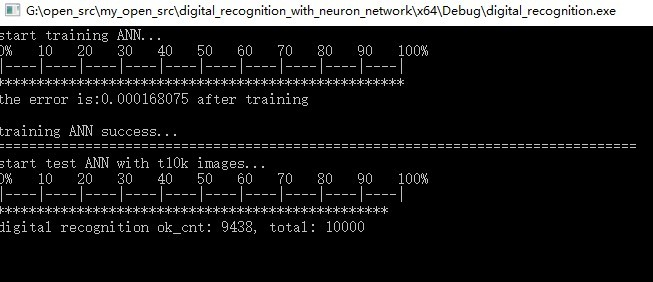[2018年8月7日更新]：在原有的基础上增加了训练和测试各自的用时时间，其结果如下所示：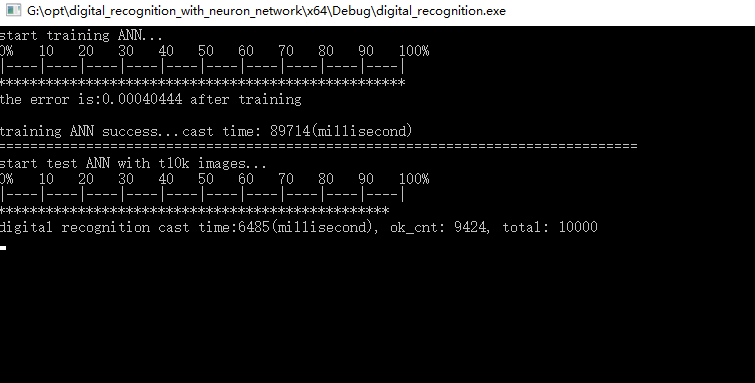07-222万+
11-074万+07-163947
04-284611
03-038032
05-311万+
07-191万+
10-204546
01-066万+